# Python基于ImageAI实现完整的流程：数据集构建、模型训练、识别预测\

♚\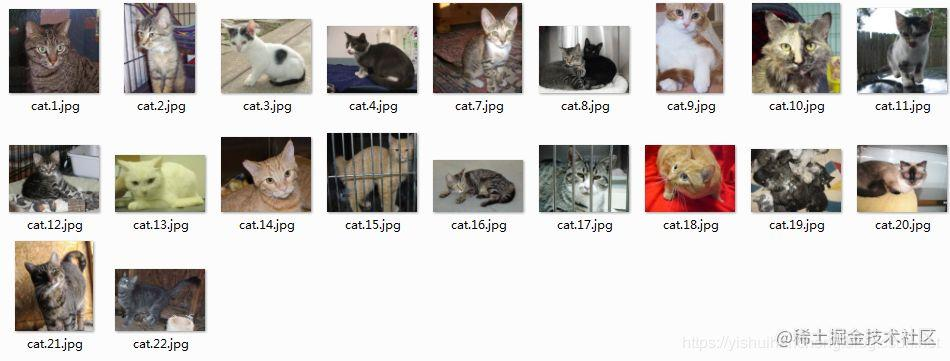``````#!usr/bin/env python# encoding:utf-8from __future__ import division"""__Author__:沂水寒城功能：Python基于ImageAI实现完成的流程：数据集构建、模型训练、识别预测"""import osimport threadingfrom imageai.Prediction import ImagePredictionfrom imageai.Prediction.Custom import ModelTrainingfrom imageai.Prediction.Custom import CustomImagePredictiondef modelTrain(dataDir='data',classNum=2,epochs=100,batch_size=32):    '''    模型训练部分    '''    #创建了ModelTraining类的新实例    model_trainer = ModelTraining()    #将模型类型设置为ResNet    model_trainer.setModelTypeAsResNet()    #设置我们想要训练的数据集的路径    model_trainer.setDataDirectory(dataDir)    #模型训练    '''    num_objects：该参数用于指定图像数据集中对象的数量    num_experiments：该参数用于指定将对图像训练的次数，也称为epochs    enhance_data（可选）：该参数用于指定是否生成训练图像的副本以获得更好的性能。    batch_size：该参数用于指定批次数量。由于内存限制，需要分批训练，直到所有批次训练集都完成为止。    show_network_summary：该参数用于指定是否在控制台中显示训练的过程。    '''    model_trainer.trainModel(num_objects=classNum, num_experiments=epochs,enhance_data=True,                              batch_size=batch_size, show_network_summary=True)    print('Model Train Finished!!!')def modelPredict(model_path='data/models/model_ex-001_acc-0.500000.h5',                 class_path='data/json/model_class.json',                 pic_path='a.jpg',classNum=2,resNum=5):    '''    模型预测部分    prediction_speed[模型加载的速度]: fast faster  fastest    '''    prediction=CustomImagePrediction()    prediction.setModelTypeAsResNet()    prediction.setModelPath(model_path)    prediction.setJsonPath(class_path)    prediction.loadModel(num_objects=classNum,prediction_speed='fastest')     predictions,probabilities=prediction.predictImage(pic_path,result_count=resNum)    for eachPrediction, eachProbability in zip(predictions, probabilities):        print(eachPrediction+" : "+str(eachProbability))if __name__=='__main__':    #模型训练    modelTrain(dataDir='data',classNum=2,epochs=10,batch_size=8)    #模型识别预测    modelPredict(model_path='data/models/model_ex-001_acc-0.500000.h5',                 class_path='data/json/model_class.json',                 pic_path='test.jpg',classNum=2,resNum=5)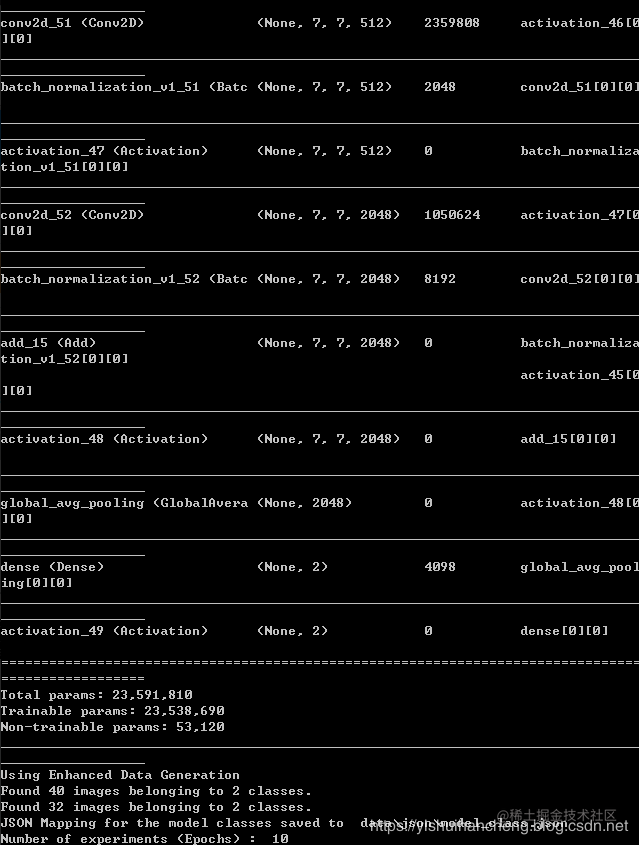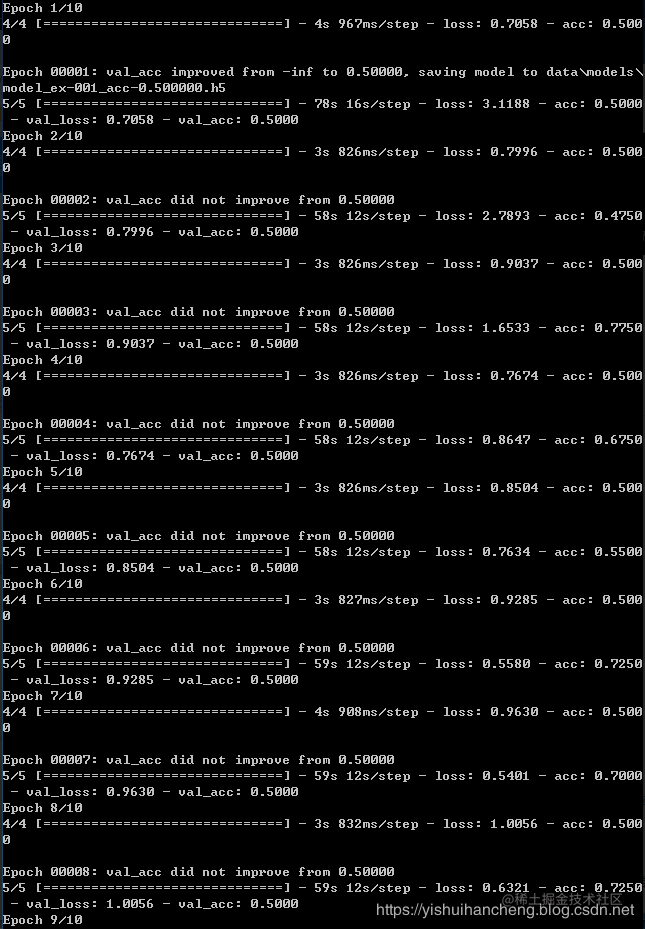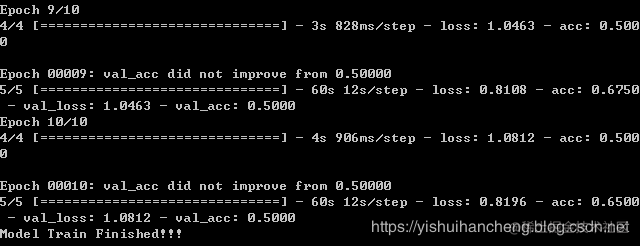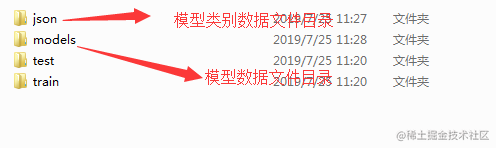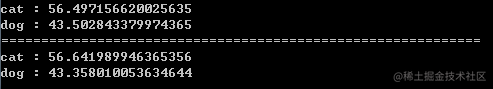\\

Python中文社区作为一个去中心化的全球技术社区，以成为全球20万Python中文开发者的精神部落为愿景，目前覆盖各大主流媒体和协作平台，与阿里、腾讯、百度、微软、亚马逊、开源中国、CSDN等业界知名公司和技术社区建立了广泛的联系，拥有来自十多个国家和地区数万名登记会员，会员来自以公安部、工信部、清华大学、北京大学、北京邮电大学、中国人民银行、中科院、中金、华为、BAT、谷歌、微软等为代表的政府机关、科研单位、金融机构以及海内外知名公司，全平台近20万开发者关注。

\▼ 点击成为社区注册会员 **「在看」**一下，一起PY！